ISSN: 2322-0066

Reach Us+44-1625-708989
All submissions of the EM system will be redirected to Online Manuscript Submission System. Authors are requested to submit articles directly to Online Manuscript Submission System of respective journal.

# The Bring-Jerrard Quintic Equation, its Algebraic Solution by Expansion

Mathematics/Physics teacher at Ngao girls, Secondary School, Kenya

*Corresponding Author:
Samuel BB
Mathematics/Physics teacher at Ngao girls
Secondary School, Kenya
E-mail: sbonayab@gmail.com

Received date: 03/06/2017; Accepted date: 14/07/2017; Published date: 05/06/2017

Visit for more related articles at Research & Reviews: Research Journal of Biology

## Abstract

I present a method of solving the Bring-Jerrard quintic equation by expansion. The aim of this research is to contribute further to the knowledge of quintic equations. The quest for a formula for the quintic equation has preoccupied mathematicians for many centuries. The general quintic equation in its trinomial form is called the Bring-Jerrard quintic equation.

The Bring – Jerrard equation has two parameters. The objective of this presentation is to present the expansion method as a viable tool for solving quintic equations and higher degree polynomials. In this research I split the unknown quantity in the quintic equation into three unknowns and expand and subsequently contract to the lowest possible form. The contraction of the expanded form involves factoring out the three unknown quantities to give the equation a solvable form. Three simultaneous equations in the three unknowns are then formed from the expanded quintic equations. The solution of the three equations yields an algebraic solution of the Bring-Jerrard quintic equation.

#### Keywords

Solving the Bring-Jerrard quintic equation by expansion, Algebraic solution of the Bring-Jerrard quintic equation, preoccupied mathematicians for centuries, Quintic equations are part of polynomial equations

#### Introduction

Background and Literature Review

The objective of this paper is to add further to the research into the solution quintic of equations that has preoccupied mathematicians for centuries.

Quintic equations are part of polynomial equations. From literature [1, 2] polynomial equations were first investigated more than four thousand years ago.

The general quintic equation takes the form(1)

Abel and Ruffini showed that proved that it impossible to solve over a field of rational numbers, Rosen 

Bring  and Jerrard  have shown that the equation can be reduced to a simple form with two parameters. That is,(2)

The equation 2 above is now called the Bring-Jerrard quintic equation, a trinomial form of the general quintic equation.

Glashan , Young , Runge  showed that some form of the Bring-Jerrard quintic equation is solvable in radicals. Spearman and William  came with similar results.

Many others contributed in various ways.

Motlotle , in his 2011 master’s thesis managed to present a formula for solving the Bring-Jerrard quintic equation using the Newton’s sum formula. In his contribution Motlotle convincingly argued that Abel’s impossibility proof has been misconstrued by many as meaning that no general algebraic solution of the quintic equation is attainable. He showed that such a formula in unattainable only within a field of Rational numbers. He then moved on to deriving a formula.

The problem is that the approach he used is too involving that it dampens any hope for coming up with similar formulae for solving higher degree polynomial equations.

In this paper the unknown x of the Bring-Jerrard quintic equation is substituted by the unknowns u, v, w, that is x=u+v+w.

The resulting expanded quintic equation is then factorized to a solvable form. Three equations are then identified from the expanded quintic equation and are used to obtain and algebraic solution of the quintic equation.

The approach used is simple and can be used to derive formulae for higher degree polynomials.

Statement of the Problem

Could there be other methods other the conventional ones that can yield an algebraic solution to the quintic equation? Could there be a viable approach by which the quintic equations can be split into some three solvable simultaneous equations? Is there a viable method by which the unknown in the algebraic equation is parameterized and subsequently put in a form such that it can degenerate to solvable forms?

If the unknown is replaced by three unknowns, what final form should the resulting expansion take to achieve an algebraic solution?

I seek to present a method of solving the Bring-Jerrard quintic equation by which three derivative and degenerate equations are solved.

#### Objectives

The main objective of this paper is to derive the algebraic solution of the quintic equation by a method in which a set of three simultaneous equations is solved.

• The specific objectives include:

• Expansion of subsequent quintic equation after replacing the unknown by three unknown parameters

• Simplifying the achieved expansion to achieve a solvable form.

• Split the simplified solvable form into three solvable equations to achieve a general solution of the quintic equation.

#### Methodology

Solution of the Cubic Equation

Before solving the quintic equation, I will attempt to derive the cubic equation using the proposed method.

Consider the cubic equation,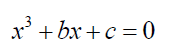(3)

If we take x=u+v 2 then,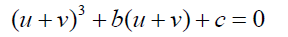(4)

The expanded form of 2 is:(5)

3 can be simplified to take the form:(6)

Take(7)

And(8)

From 0.5, b = −3uv (9)

Substituting 6 into 7 and simplifying:(10)

One of the roots of 8 is(11)

Similarly it can be shown that one of the roots of v is given by:(12)

One root of the above cubic equation is given by,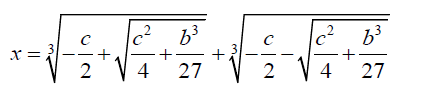(13)

The above method can be extended to provide a solution to the Bring-Jerrard quintic equation.

Solution of the Bring-Jerrard Quintic Equation

Consider the Bring-Jerrard quintic equation below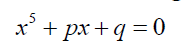(14)

If we take x = u + v + w then,

( u + v + w)5 + b( u + v + w ) + c = 0 (15)

The expanded form of 3 is:(16)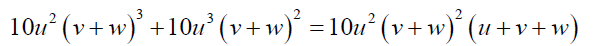(17)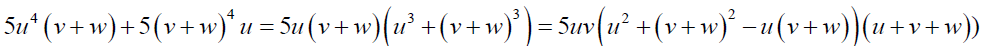(18)

Substituting 5 and 6 into 4(19)

0.7 can further be simplified to:(20)

8 can further be simplified to:(21)

The equation 9 can be changed to three equations with three unknown quantities u, v and w.

Take:(22)

That is:(23)

and(24)

The form the equation 11 is in cannot generate an algebraic solution for the quintic equation.

Two useful forms are:(25)

If(26)

We can now introduce the third equation to make the quintic equation solvable. That is if we take:

s2 − t2 = u (27)

Then substation of 15 into 11 gives the result:

b = −5u2 (v + w) , or(28)

This makes the quintic equation solvable

Substituting 16 into 12 and simplifying;(29)

Then equation 17 takes the form:(30)(31)

The equation 18 reduces to:(32)

One of the roots of 20 is given by:(33)

From 13, 14 and 15:

( u + v + w)2 - u( v + w )= u (34)

The expansion of 2.3 is

( v + w)2 + u( v + w )+ u2-u=0(35)

Substituting 22 into 24(36)

sTwo of the roots of the quintic equation are:(37)

We note from 13

s = x

The other roots are obtained by solving the subsequent auxiliary equations.

Using equations 13, 14 and 15 we can calculate the unknown w:(38)

Summary Recommendations And Conclusion

If the unknown quantity in the Bring-Jerrard quintic equation is replaced by three parameters and the resulting expansion  simplified to a solvable form it is possible to arrive at an algebraic solution. The algebraic solution is arrived at by solving three simultaneous equation  split from the simplified expanded equation. From this treatise it can be concluded that a radical or algebraic solution of the quintic equation is achievable. Radical solution is loosely taken to mean root form. The research contests t he generally acclaimed notion proposed in Abel-Ruffini theorem and expounded in Goalis Theory that quantic equations in general Are algebraically indeterminate. The Abel-Ruffini theorem is incomplete since it didn`t put into consideration the broad spectrum of mathematical possibilities in the for algebraic solution of higher degree polynomials.

I recommend a rewrite of Galois Theory to take into account the current reality. The current reality is that Quintic equations are in general solvable.